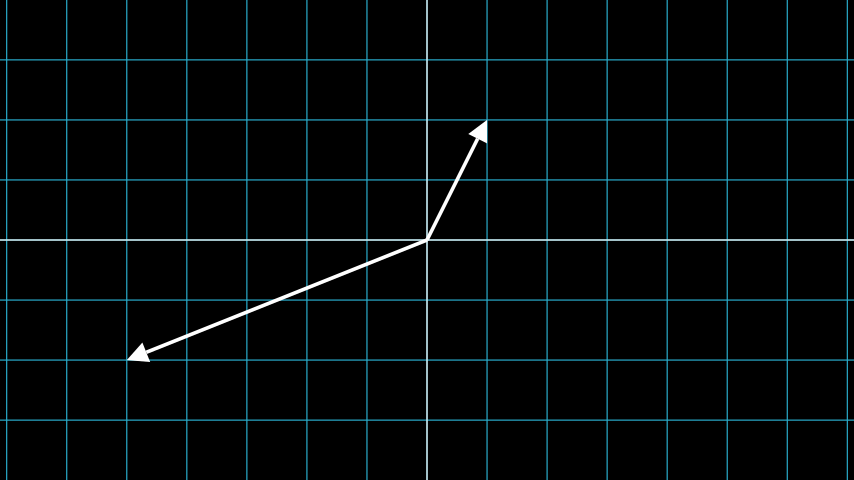# Vector¶

Qualified name: `manim.mobject.geometry.Vector`

class Vector(direction=array([1., 0., 0.]), buff=0, **kwargs)[source]

A vector specialized for use in graphs.

Parameters
• direction (Union[`list`, `numpy.ndarray`]) – The direction of the arrow.

• buff (`float`) – The distance of the vector from its endpoints.

• kwargs (Any) – Additional arguments to be passed to `Arrow`

Examples

Example: VectorExample```from manim import *

class VectorExample(Scene):
def construct(self):
plane = NumberPlane()
vector_1 = Vector([1,2])
vector_2 = Vector([-5,-2])
```

Methods

 `coordinate_label` Creates a label based on the coordinates of the vector.

Attributes

 `animate` Used to animate the application of a method. `animation_overrides` `depth` The depth of the mobject. `height` The height of the mobject. `width` The width of the mobject.
coordinate_label(integer_labels=True, n_dim=2, color='#FFFFFF')[source]

Creates a label based on the coordinates of the vector.

Parameters
• integer_labels (bool) – Whether or not to round the coordinates to integers.

• n_dim (int) – The number of dimensions of the vector.

• color (str) – The color of the label.

Examples